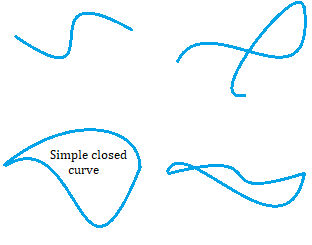# Simple Closed Curve

A simple closed curve is closed but does not intersect itself at any point. You could call it a non-self-intersecting continuous loop. A Familiar example is a circle.

It’s called closed because its first and last points are the same. It’s “simple” because it has no repeated points other than, perhaps, the first (which is equal to the last).

Simple closed curves are also known as Jordan curves. They are named after mathematician Camille Jordan (1838-1922), who first proved a groundbreaking theorem known as the Jordan curve theorem. This theorem tells us that every simple closed curve divides the plane to an interior region and an exterior region, and every continuous path connecting a point of one of these to a point of the other will intersect the curve somewhere. Although this might seem simple and obvious, it is actually very difficult to prove.

## Examples of Simple Closed Curve

A circle is perhaps the simplest example of a Jordan curve. But squashed circles and other irregular shapes can be Jordan curves too, just so long as their sides do not intersect each other and they are closed. A square is a Jordan curve. So is a rectangle.

Of the four images below, only the bottom left is a simple closed curve. The one beside it on the right is also closed, but it isn’t simple. The two on top are not closed.## Properties of Simple Closed Curve

Jordan curves are topologically equivalent to a unit circle (a circle with a radius of 1). If you take any triangle T and a simple closed curve C, C will have an inscribed triangle similar to the triangle T.

## References

Tverberg, Helge (1980), “A proof of the Jordan curve theorem” (PDF), Bulletin of the London Mathematical Society, 12 (1): 34–38, CiteSeerX 10.1.1.374.2903, doi:10.1112/blms/12.1.34. Retrieved from https://londmathsoc.onlinelibrary.wiley.com/doi/abs/10.1112/blms/12.1.34 on August 18, 2019
Szabo, Tibor. Graph Theory– WS 06/07 Lecture Notes. Lecture 13, Turán-Type Problems, Planar Graphs I. Published Jan 24, 2007. Swiss Federal Institute of Technology, Zurich. Retrieved from https://www.ti.inf.ethz.ch/ew/courses/GT06/lectures/PDF/lecture13.pdf on August 18, 2019

CITE THIS AS:
Stephanie Glen. "Simple Closed Curve" From StatisticsHowTo.com: Elementary Statistics for the rest of us! https://www.statisticshowto.com/simple-closed-curve/Need help with a homework or test question? With Chegg Study, you can get step-by-step solutions to your questions from an expert in the field. Your first 30 minutes with a Chegg tutor is free!